# Regularization of sequences

(diff) ← Older revision | Latest revision (diff) | Newer revision → (diff)

Let,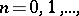be a sequence of real numbers (indexed by the non-negative integers). A regularization of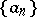is a sequence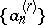obtained fromby replacing certainwhich are "excessively high" with respect to the others by suitable lower values. An important application of regularized sequences is to the problem of equivalence of classes of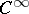-functions; that is, the problem of when two sequences of constants determine the same quasi-analytic class of functions. The answers tend to be given in the form that the two sequences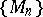and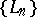determine the same quasi-analytic class if suitably regularized sequences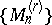and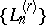are the same, cf. [a1], [a2].

Some important regularization procedures are as follows. A sequenceof real numbers is called a convex sequence if the function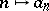is convex, i.e. if for all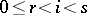,that is, if the point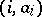is located below or on the segment in the plane joiningand(cf. Convex function (of a real variable)).

The convex regularization, or Newton regularization,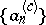ofis the largest convex minorant of(cf. Majorant and minorant, 1)).

The log-convex regularization, or convex regularization by means of the logarithm, of a sequence of positive numbersis the sequence of positive numberssuch that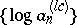is the convex regularization of. It is defined by the relations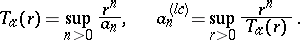The exponential regularizationofis defined by the relations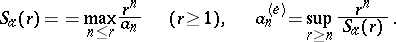The Newton regularization of a sequenceis very much related to the Newton polygon of(this explains the name "Newton regularization" , cf. also Newton diagram, which discusses the context in which the Newton polygon first arose). For a finite sequence, its Newton polygon is the highest convex polygonal line in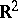joining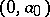to, i.e. it is the polygonal line consisting of the segments joiningto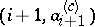,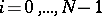. Thus, the number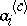is the ordinate of the point of the Newton polygon ofwith abscissa.

An example of this for the sequence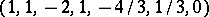,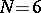, with convex regularization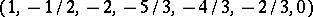, is given in Fig. a.Figure: r080940a

To avoid certain pathologies (like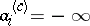for all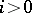), letbe bounded from below. The Newton polygon of this sequence is defined as the limit of the Newton polygons of the finite sequences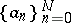as. It remains true thatis determined by the condition thatlies on the Newton polygon of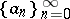.

Letbe a non-Archimedean valued field with valuation(cf. also Norm on a field). Let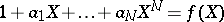be a polynomial of degreeover. The Newton polygon of the polynomial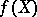is the Newton polygon of the sequence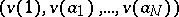. It carries immediate information on the valuations of the roots of(in a complete algebraic closure of). Indeed, ifis the slope of a segment of the Newton polygon of (abscissa) length, then there are preciselyroots of valuation(counted with multiplicities); an analogous result holds for roots of power series (this is related to a-adic Weierstrass preparation theorem, cf. (the editorial comments to) Weierstrass theorem, and [a3]).

The Newton polygon of a sequencecan be obtained geometrically as follows. For all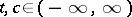one considers the lineinthrough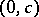of slope; it is given by the equation. Letbe the supergraph of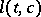. Letbe the graph of,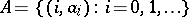. Then the Newton polygon is the lower boundary of the convex setAs noted, the Newton regularization (convex regularization) of a sequenceis determined by its Newton polygon. This construction has been generalized. Let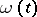be a non-decreasing function ofwith values in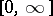. Let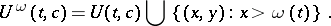The lower boundary of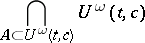now defines the-regularized sequence. Newton and exponential regularization correspond to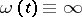and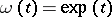, respectively.

How to Cite This Entry:
Regularization of sequences. Encyclopedia of Mathematics. URL: http://encyclopediaofmath.org/index.php?title=Regularization_of_sequences&oldid=19031